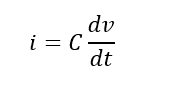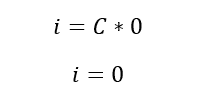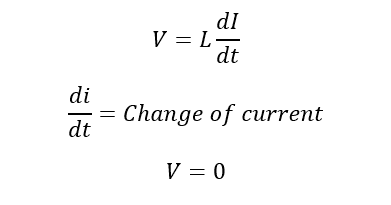# Why DC Does Not Have Power Factor ?

### Why DC Does Not Have Power factor:

In AC Power system power factor plays major role while using AC loads. Here power factor is nothing but cosine of voltage and current. generally, AC loads consist of resistor, inductor and capacitor. Remember that, alternating current means the current changes its polarity periodically. In that, resister only oppose the flow of electron in terms of voltage drops and current flow through it. But inductive loads and capacitive loads are opposing the rate of change of current (inductive) and voltage (capacitive). Since the opposition is measured in terms of angle. The cosine of these opposition is called power factor.

But in DC let see the response of capacitor and inductor:

### Capacitor:

The current flow through the capacitor is I, C is the capacitance of the capacitor and the voltage across capacitor is dv/dt. Therefore, the current i isIn DC the rate of change of voltage is zero, dv/dt=0 Since the DC does not change its voltage.. thereforeThe current flow through the capacitor is zero. So that, In DC source the capacitor act as open circuit. Therefore, open circuit does not have power factor.

### Inductor:

Consider I is the current flow through an inductor circuit is DC, then the voltage V across the inductor is..But dt/dt=0 since the rate of change of current in dc is zero.

The Voltage across the inductor is zero, the voltage across any component is zero which means the resistance is zero, the inductor act as short circuit.

Here you can see that the capacitor and inductor act as open circuit and short circuit. For open circuit and short circuit power factor cannot be calculated. The remaining one is resister, you know resistance power factor is one. That’s why we neglect the power factor in DC circuits.